# 曲線：內插和控制點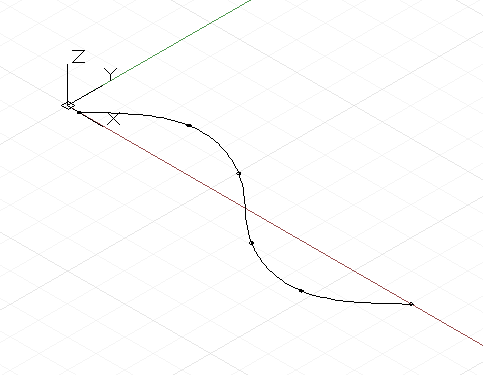``````num_pts = 6;

s = Math.Sin(0..360..#num_pts) * 4;

pts = Point.ByCoordinates(1..30..#num_pts, s, 0);

int_curve = NurbsCurve.ByPoints(pts);
``````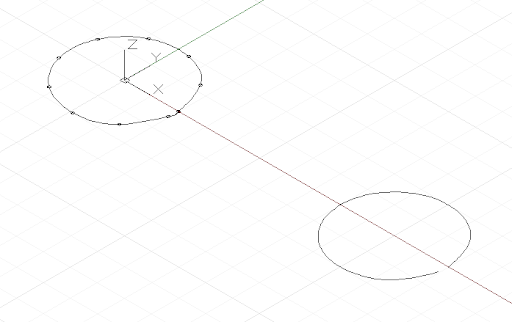``````pts = Point.ByCoordinates(Math.Cos(0..350..#10),
Math.Sin(0..350..#10), 0);

// create an closed curve
crv = NurbsCurve.ByPoints(pts, true);

// the same curve, if left open:
crv2 = NurbsCurve.ByPoints(pts.Translate(5, 0, 0),
false);
``````

NurbsCurve 以幾乎相同的方式產生，輸入點代表直線段的端點，第二個參數稱為程度，指定平滑化曲線的量和類型。* 程度 1 的曲線沒有平滑化；它是一條聚合線。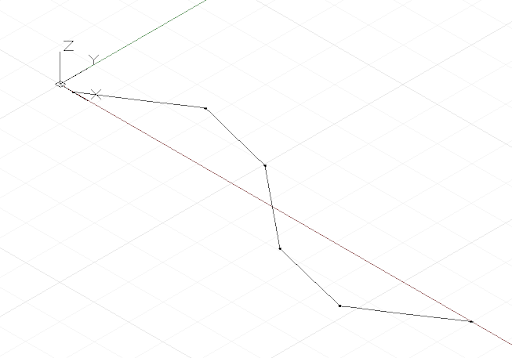``````num_pts = 6;

pts = Point.ByCoordinates(1..30..#num_pts,
Math.Sin(0..360..#num_pts) * 4, 0);

// a B-Spline curve with degree 1 is a polyline
ctrl_curve = NurbsCurve.ByControlPoints(pts, 1);
``````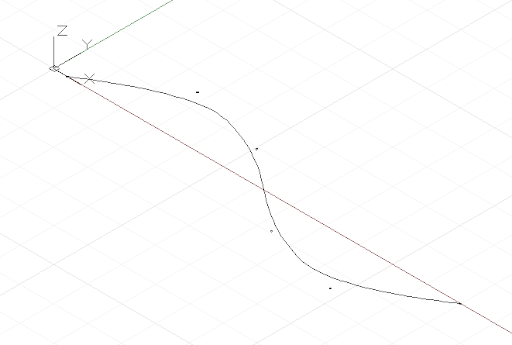``````num_pts = 6;

pts = Point.ByCoordinates(1..30..#num_pts,
Math.Sin(0..360..#num_pts) * 4, 0);

// a B-Spline curve with degree 2 is smooth
ctrl_curve = NurbsCurve.ByControlPoints(pts, 2);
``````

Dynamo 支援最高到程度 20 的 NURBS (非均勻的合理 B 雲形線) 曲線，以下腳本說明增加平滑程度對曲線造型的影響：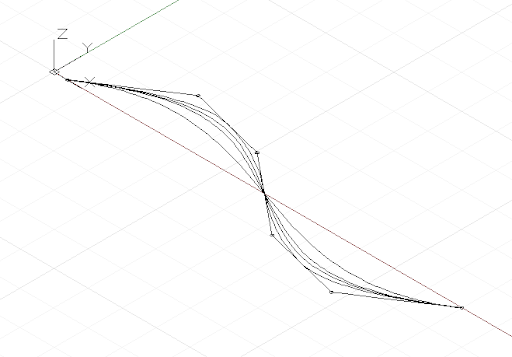``````num_pts = 6;

pts = Point.ByCoordinates(1..30..#num_pts,
Math.Sin(0..360..#num_pts) * 4, 0);

def create_curve(pts : Point[], degree : int)
{
return = NurbsCurve.ByControlPoints(pts,
degree);
}

ctrl_crvs = create_curve(pts, 1..11);
``````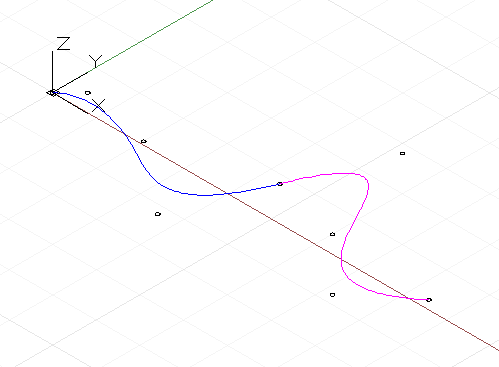``````pts_1 = {};

pts_1 = Point.ByCoordinates(0, 0, 0);
pts_1 = Point.ByCoordinates(1, 1, 0);
pts_1 = Point.ByCoordinates(5, 0.2, 0);
pts_1 = Point.ByCoordinates(9, -3, 0);
pts_1 = Point.ByCoordinates(11, 2, 0);

crv_1 = NurbsCurve.ByControlPoints(pts_1, 3);

pts_2 = {};

pts_2 = pts_1;
end_dir = pts_1.Subtract(pts_1.AsVector());

pts_2 = Point.ByCoordinates(pts_2.X + end_dir.X,
pts_2.Y + end_dir.Y, pts_2.Z + end_dir.Z);

pts_2 = Point.ByCoordinates(15, 1, 0);
pts_2 = Point.ByCoordinates(18, -2, 0);
pts_2 = Point.ByCoordinates(21, 0.5, 0);

crv_2 = NurbsCurve.ByControlPoints(pts_2, 3);
``````
• 這是一個非常簡化的 NURBS 曲線幾何圖形說明，如需更準確更詳細的討論，請參閱參考資料中的 Pottmann, et al, 2007。